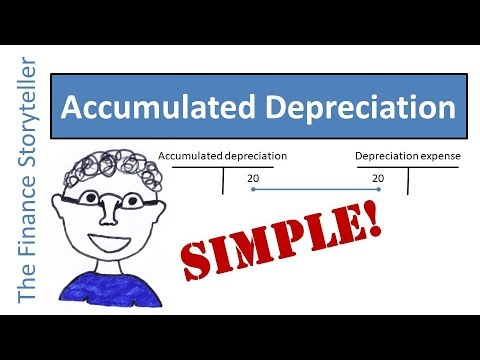# Accumulated depreciation

12
45What is accumulated depreciation? Accumulated Depreciation is the title of the contra asset account on the balance sheet which is used when depreciation expense is recorded each accounting period. Let’s review how accumulated depreciation works with an example.

Let’s take a fixed asset which we purchased for \$100,000 and use for 5 years. When we bought the fixed asset, we put it on the balance sheet for its full value. When we start using it, assuming we use linear depreciation and a residual value of 0, we book a cost of \$20,000 per year as a debit in our income statement (or P&L), and the offset of that journal entry is a credit to a balance sheet account called accumulated depreciation. If you want to know the book value or carrying value of the fixed asset at the end of year 1, you net together the historical cost of the fixed asset on the balance sheet, and the accumulated depreciation on the balance sheet, which is \$100,000 minus \$20,000, so the net book value equals \$80,000. This information is recorded in two separate accounts, as you want to preserve the information of what you originally spent to purchase the asset, and what you have depreciated on it history-to-date. In year 2, you repeat the same depreciation journal entry, and get to a book value of \$60,000. And on and on for years 3, 4 and 5.

The difference between depreciation expense and accumulated depreciation is that depreciation expense appears as an expense on the income statement, and accumulated depreciation is a contra asset reported on the balance sheet. The income statement is reset to zero at the start of every year, so you start with zero in depreciation expense every year, and build it up in twelve monthly increments. The accumulated depreciation amount on the balance sheet keeps growing over the years, all the way to the point that the fixed asset is fully depreciated, or the point where you sell the fixed asset at, above or below book value.

Philip de Vroe (The Finance Storyteller) aims to make strategy, finance and leadership enjoyable and easier to understand. Learn the business vocabulary to join the conversation with your CEO at your company. Understand how financial statements work in order to make better stock market investment decisions. Philip delivers training in various formats: YouTube videos, classroom sessions, webinars, and business simulations. Connect with me through Linked In!

Nguồn: https://sangiaodichtienao.com

Xem thêm bài viết khác: https://sangiaodichtienao.com/tong-hop

1.The Finance Storyteller

Enjoyed the video? Then subscribe to the channel, and watch the video on contra accounts next: https://www.youtube.com/watch?v=ixkdvOT7ZDI

2. What happens in Year 6 when the item is fully depreciated and cannot be sold? Will the balance sheet show forever 100 as a fix asset and 100 in accumulated depreciation?

3.Manjunath Jagadish Babu

Depreciation a/c Dr.
To Accumulated Depreciation a/c.
Treatment of accounts:
1. Accumulated Depreciation
Credit balance, parked as a liability.
2. Depreciation a/c
Nominal account. Balance written off to P&L a/c.
P&L a/c Dr.
To Depreciation a/c

4. so accumulated depreciation and depreciation in the profit loss and income statement will be the same number right ( if no b/f for the accumulated depreciation) ?

5. Literally no one can understand you

6. can you do a video on decreasing in accumulated depreciation and selling the asset?

7. Thank u so much💜

8. Thank you

9.Diontae Daughtry

Thank you this was very helpful and informative 👍👍

10. Quick simple and spot on

11. Thanks count dracula

12. The cost of the fixed asset is \$100,000, provision for depreciaiton is \$10,000,

depreciation charge for the year are \$2,000. Show the net book value of the fixed

asset in the Balance sheet

A \$74,000

B \$55,000

C \$24,000

D \$78,000

sir I found answer d 78000 but how I don't know sir please explain.